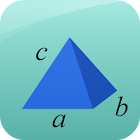Area and Volume Calculator

All Android applications categories

All Android games categories# Area and Volume Calculator

by: 2.2K 7.8

7.8 Users
rating

## Screenshots

Description

This free app is a geometric calculator which is able to calculate area and volume.

Area Calculator: You are able to calculate the area for the most important geometric figures. You can calculate the area of circle, ellipse, rectangle, square, trapezoid, triangle, parallelogram, rhombus, sector and triangle.

Volume Calculator: You are able to calculate the volume for the most important geometric objects. You can calculate the volume of cone, cube, cuboid, cylinder, sphere, square pyramid and tetrahedron.

Best math tool for school and college! If you are a student, it will help you to learn geometry!

Tags: moisesfurbinosoares , application pour aire et volume objets , calculadoras online para volumen de tetraedro

## Users review

from 2.158 reviews

"Great"

7.8